Author: Oscar Cronquist Article last updated on May 05, 2022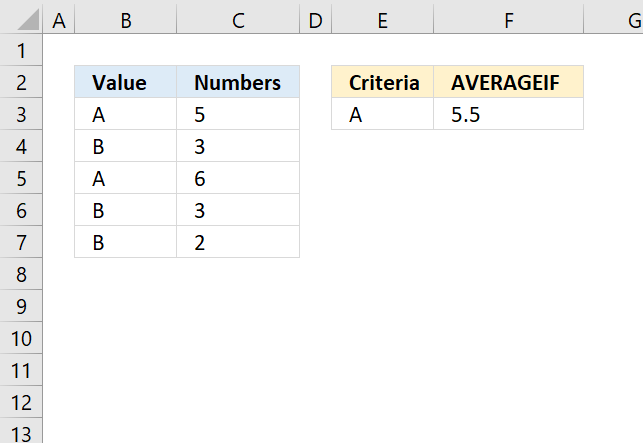The AVERAGEIF function returns the average of cell values that are valid for a given condition.

Formula in cell D3:

=AVERAGEIF(B3:B7,E3,C3:C7)

The AVERAGEIF function is available for Excel 2010 users and later versions.

## 1. Excel Function Syntax

AVERAGEIF(range, criteria, [average_range])

## 2. Arguments

 range Required. One or more cells to average, including numbers or names, arrays, or references that contain numbers. criteria Required. A cell reference, expression or text that determines which values to be evaluated. average_range Optional. Cells to average. If not entered, range is used.

## 3. Which values are excluded?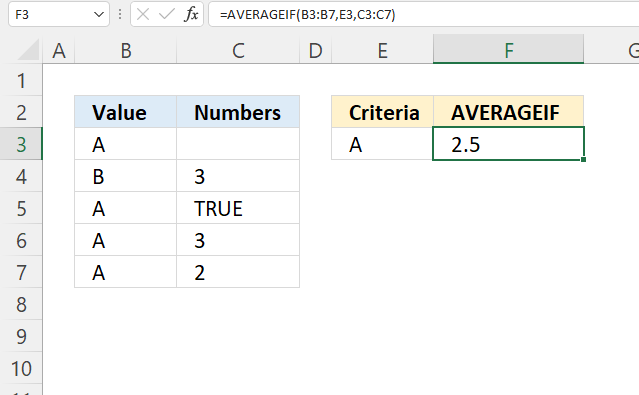Boolean values TRUE or FALSE are excluded in the calculation, see cell C5 in the image above.

An empty cell in argument average_range is excluded, see cell C3 in the picture above.

If a condition in argument average_range is empty is the same as 0 (zero).

## 4. AVERAGEIF Function not working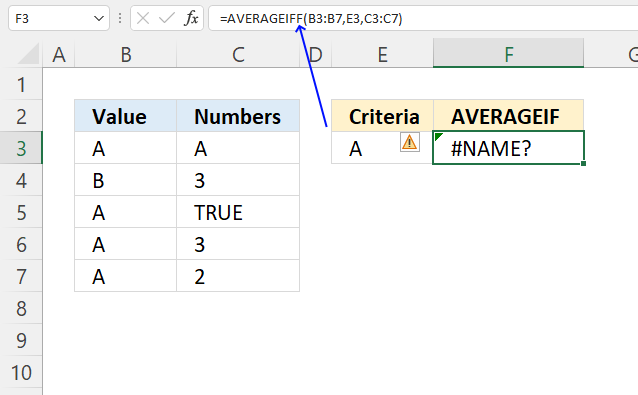The AVERAGEIF function returns #DIV0! if no values match the criteria. A #NAME error translates to a misspelled function in your formula. The AVERAGEIF function ignores text and boolean values, however, not error values.

Trying to use an asterisk or question mark as a condition? It won't work as they are wildcard characters, however, there is a workaround. Use the ~ (tilde) character to escape wildcard characters.

## 5. How to use wildcard characters in the AVERAGEIF function

It is possible to use wildcard characters like (?) question mark or (*) asterisk.

(?) question mark matches any single character.

(*) asterisk matches any sequence of characters even zero charcaters.

Use a tilde (~) before to escape the wildcard characters meaning finding the actual question mark or asterisk.

## 5.1 AVERAGEIF function - begins with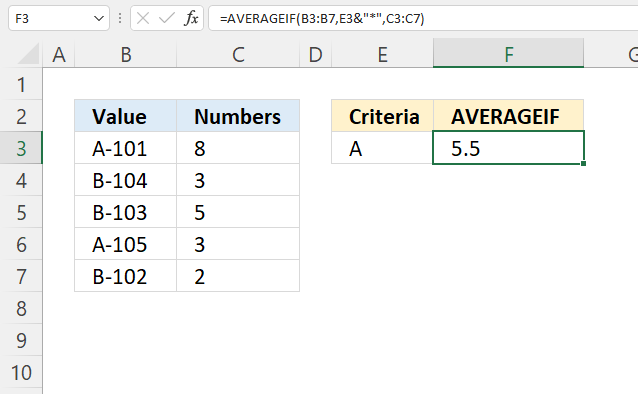The image above demonstrates how to average corresponding numbers if a value on the same row begins with a specific condition.

Formula in cell F3:

=AVERAGEIF(B3:B7, E3&"*", C3:C7)

### Explaining formula

#### Step 1 - Populate arguments

AVERAGEIF(range, criteria, [average_range])

range - B3:B7
criteria - E3&"*"
[average_range] - C3:C7

#### Step 2 - Evaluate AVERAGEIF function

AVERAGEIF(B3:B7, E3&"*", C3:C7)

becomes

AVERAGEIF({"A-101"; "B-104"; "B-103"; "A-105"; "B-102"}, "A*", {8; 3; 5; 3; 2})

and returns 5.5

8 + 3 = 11

11/2 equals 5.5

## 5.2 AVERAGEIF function - ends with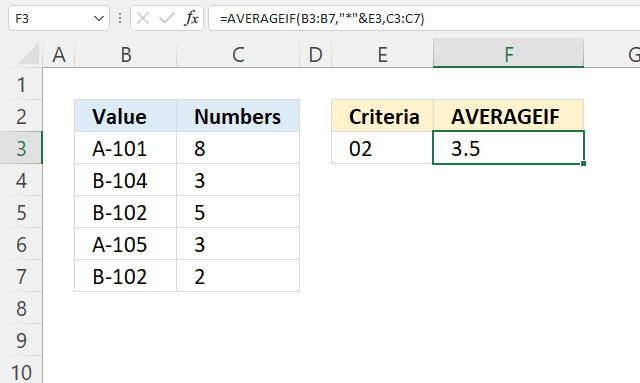The image above shows a formula in cell F3 that calculates an average if the values in cell range B3:B7 ends with a given condition specified in cell E3.

The corresponding number on the same row in cell range C3:C7 is included in the calculation if the value meets the condition.

Cells B5 and B7 meet the condition, corresponding cells are C5 and C7. They contain 5 and 2. 5 + 2 equals 7. 7 / 2 equals 3.5

Formula in cell F3:

=AVERAGEIF(B3:B7, "*"&E3, C3:C7)

### Explaining formula

#### Step 1 - Populate arguments

AVERAGEIF(range, criteria, [average_range])

range - B3:B7
criteria - "*"&E3
[average_range] - C3:C7

#### Step 2 - Evaluate AVERAGEIF function

AVERAGEIF(B3:B7, "*"&E3, C3:C7)

becomes

AVERAGEIF({"A-101"; "B-104"; "B-102"; "A-105"; "B-102"}, "*02", {8; 3; 5; 3; 2})

and returns 3.5

5 + 2 = 7

7/2 equals 3.5

## 5.3 AVERAGEIF function - contains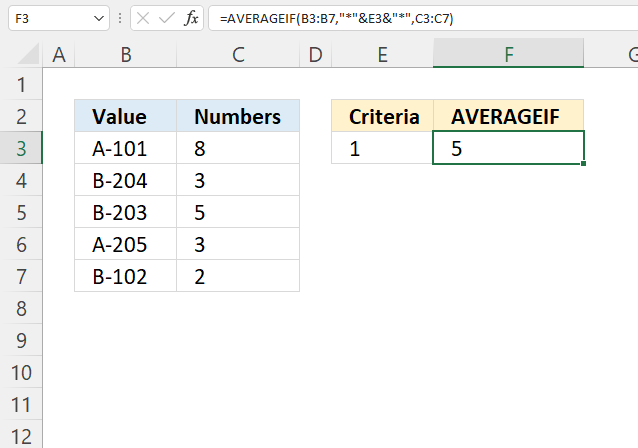Formula in cell F3:

=AVERAGEIF(B3:B7, "*"&E3&"*", C3:C7)

### Explaining formula

#### Step 1 - Populate arguments

AVERAGEIF(range, criteria, [average_range])

range - B3:B7
criteria - "*"&E3&"*"
[average_range] - C3:C7

#### Step 2 - Evaluate AVERAGEIF function

AVERAGEIF(B3:B7, "*"&E3&"*", C3:C7)

becomes

AVERAGEIF({"A-101"; "B-204"; "B-203"; "A-205"; "B-102"}, "*1*", {8; 3; 5; 3; 2})

and returns 5.

8 + 2 = 10

10/2 equals 5

## 5.4 AVERAGEIF function - match a specific number of characters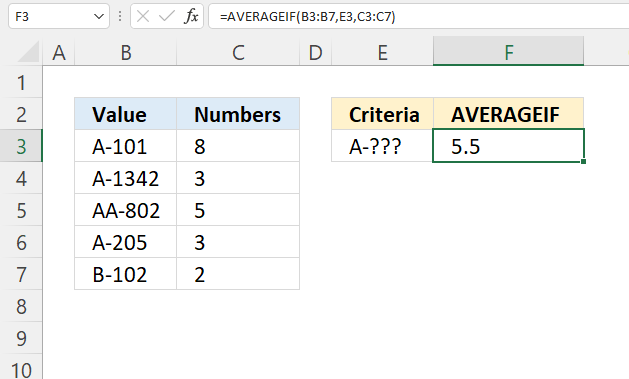The question mark character ? lets you match any single character in the condition string. The above image demonstrates a formula that uses the following criteria "A-???"

It matches values in cells B3 and B6. Cell B4 contains a value that almost match, however, it is longer than the requirements. Remember that the question mark only matches a single character.

Formula in cell F3:

=AVERAGEIF(B3:B7, E3, C3:C7)

### Explaining formula

#### Step 1 - Populate arguments

AVERAGEIF(range, criteria, [average_range])

range - B3:B7
criteria - E3
[average_range] - C3:C7

#### Step 2 - Evaluate AVERAGEIF function

AVERAGEIF(B3:B7, E3, C3:C7)

becomes

AVERAGEIF({"A-101"; "A-1342"; "AA-802"; "A-205"; "B-102"}, "A-???", {8; 3; 5; 3; 2})

and returns 5.5

8 + 3 = 11

11/2 equals 5.5

## 6. Is the criteria argument in the AVERAGEIF function case sensitive?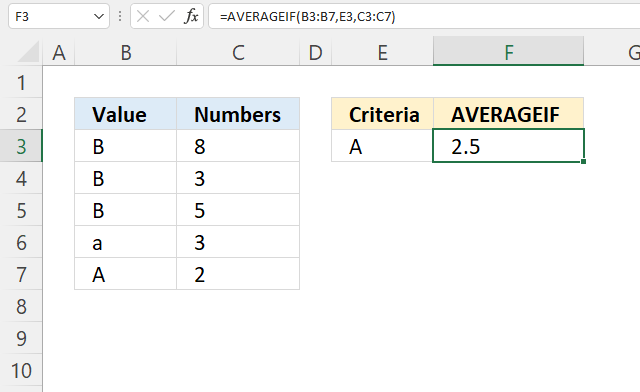Does the AVERAGEIF function differentiate between upper and lower letters?
No, the image above shows that it's not. Here is how to: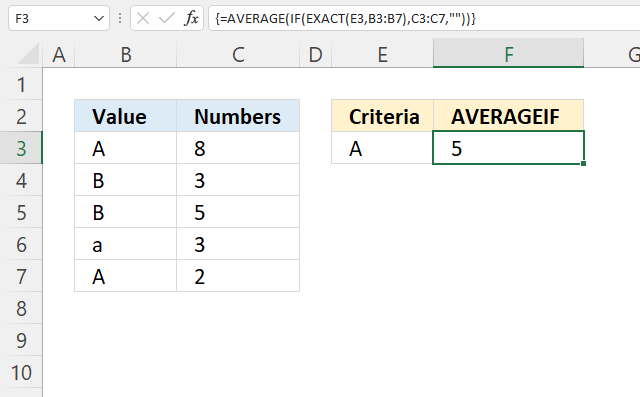Array formula in cell F3:

=AVERAGE(IF(EXACT(E3,B3:B7),C3:C7,""))

### How to enter an array formula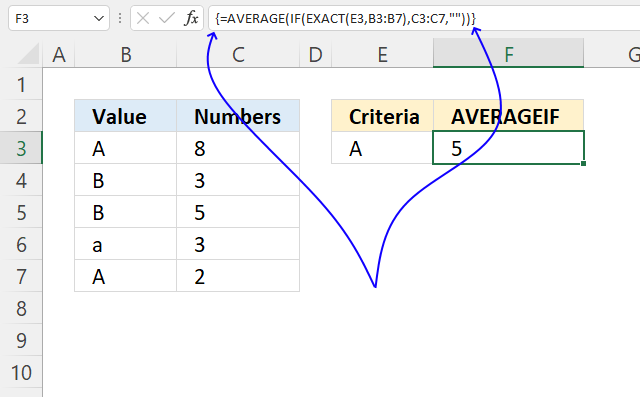1. Doublepress with left mouse button on cell F3, the prompt appears.
2. Type or paste the array formula.
3. Press and hold CTRL + SHIFT keys simultaneously.
4. Press Enter once.
5. Release all keys.

The formula is now entered as an array formula, the formula begins with and ends with a curly bracket, see the image above.

Don't enter these characters yourself, they appear automatically.

### Explaining formula

#### Step 1 - Case sensitive comparison

The EXACT function returns TRUE if two values match also considering upper and lower letters.

EXACT(value1, value2)

EXACT(E3, B3:B7)

becomes

EXACT("A",{"A";"B";"B";"a";"A"})

and returns

{TRUE; FALSE; FALSE; FALSE; TRUE}.

#### Step 2 - Replace TRUE with corresponding value

The IF function returns one value if the logical test is TRUE and another value if the logical test is FALSE.

IF(logical_test, [value_if_true], [value_if_false])

IF(EXACT(E3, B3:B7), C3:C7, "")

becomes

IF({TRUE; FALSE; FALSE; FALSE; TRUE}, C3:C7, "")

becomes

IF({TRUE; FALSE; FALSE; FALSE; TRUE}, {8; 3; 5; 3; 2}, "")

and returns

{8; ""; ""; ""; 2}.

#### Step 3 - Calculate average

The AVERAGE function calculates the average of numbers in a cell range.

AVERAGE(number1[number2], ...)

AVERAGE(IF(EXACT(E3,B3:B7),C3:C7,""))

becomes

AVERAGE({8; ""; ""; ""; 2})

and returns 5.

8 + 2 = 10

10/2 = 5

## 7. AVERAGEIF function - comparison operators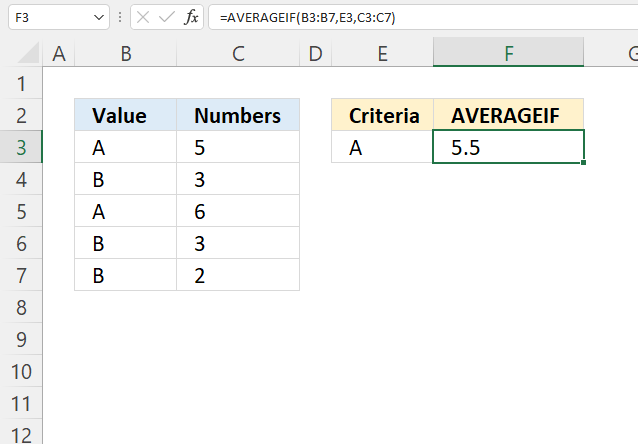You are allowed to use the following comparison operators:

• < less than character
• > larger than character
• <> not equal to
• <= less than or equal to
• >= larger than or equal to

The equal sign is never used alone with the value in the criteria argument, the image above shows how to use a condition specified in cell E3 in the AVERAGEIF function.

## 7.1 AVERAGEIF function - larger than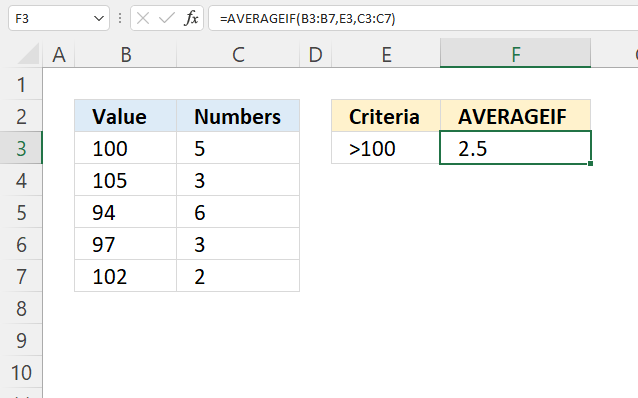Formula in cell F3:

=AVERAGEIF(B3:B7, E3, C3:C7)

### Explaining formula

#### Step 1 - Populate arguments

AVERAGEIF(range, criteria, [average_range])

range - B3:B7
criteria - E3
[average_range] - C3:C7

#### Step 2 - Evaluate AVERAGEIF function

AVERAGEIF(B3:B7, E3, C3:C7)

becomes

AVERAGEIF({100;105;94;97;102},">100",{5;3;6;3;2})

and returns 2.5

3 + 2 = 5

5/2 equals 2.5

## 7.2 AVERAGEIF function - smaller than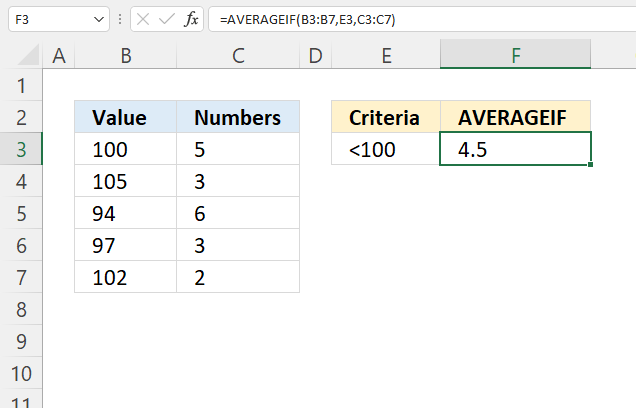Formula in cell F3:

=AVERAGEIF(B3:B7, E3, C3:C7)

### Explaining formula

#### Step 1 - Populate arguments

AVERAGEIF(range, criteria, [average_range])

range - B3:B7
criteria - E3
[average_range] - C3:C7

#### Step 2 - Evaluate AVERAGEIF function

AVERAGEIF(B3:B7, E3, C3:C7)

becomes

AVERAGEIF({100;105;94;97;102},"<100",{5;3;6;3;2})

and returns 2.5

6 + 3 = 9

9/2 equals 4.5

## 7.3 AVERAGEIF function - not equal to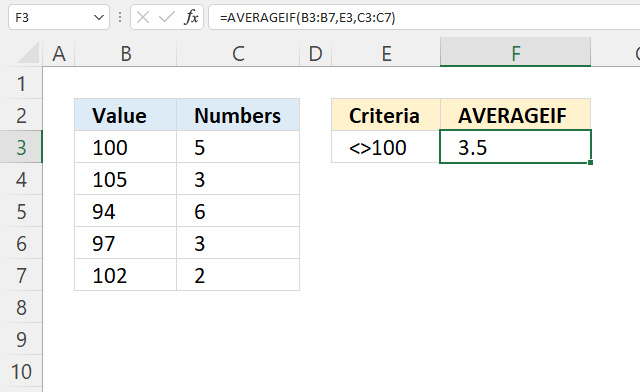Formula in cell F3:

=AVERAGEIF(B3:B7, E3, C3:C7)

### Explaining formula

#### Step 1 - Populate arguments

AVERAGEIF(range, criteria, [average_range])

range - B3:B7
criteria - E3
[average_range] - C3:C7

#### Step 2 - Evaluate AVERAGEIF function

AVERAGEIF(B3:B7, E3, C3:C7)

becomes

AVERAGEIF({100;105;94;97;102},"<>100",{5;3;6;3;2})

and returns 2.5

3 + 6 + 3 + 2 = 14

14/4 equals 3.5